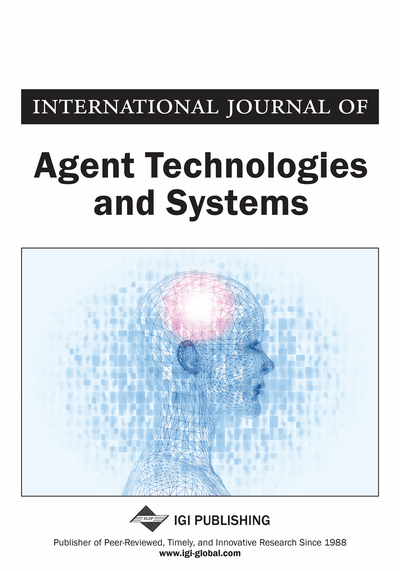# Quantifying Complexity in Networks: The von Neumann Entropy

Filippo Passerini (Perimeter Institute for Theoretical Physics, Canada) and Simone Severini (University of Waterloo, Canada)
DOI: 10.4018/jats.2009071005

## Abstract

The authors introduce a novel entropic notion with the purpose of quantifying disorder/uncertainty in networks. This is based on the Laplacian and it is exactly the von Neumann entropy of certain quantum mechanical states. It is remarkable that the von Neumann entropy depends on spectral properties and it can be computed efficiently. The analytical results described here and the numerical computations lead us to conclude that the von Neumann entropy increases under edge addition, increases with the regularity properties of the network and with the number of its connected components. The notion opens the perspective of a wide interface between quantum information theory and the study of complex networks at the statistical level.
Article Preview
Top

## The Von Neumann Entropy

The state of a quantum mechanical system with a Hilbert space of finite dimension n is described by a density matrix. Each density matrix ρ is a positive semidefinite matrix with Tr(ρ) = 1. Here we consider a matrix representation based on the combinatorial Laplacian to associate graphs to specific density matrices.

Let G = (V, E) be a simple undirected graph with set of vertices V(G) = {1, 2, …,n} and set of edges. The adjacency matrix of G is denoted by A(G) and defined by [A(G)]u,v = 1 if {u, v} ∈ E(G) and [A(G)]u,v = 0, otherwise.

## Complete Article List

Search this Journal:
Reset
Open Access Articles: Forthcoming
Volume 9: 1 Issue (2017)
Volume 8: 1 Issue (2016)
Volume 7: 3 Issues (2015)
Volume 6: 4 Issues (2014)
Volume 5: 4 Issues (2013)
Volume 4: 4 Issues (2012)
Volume 3: 4 Issues (2011)
Volume 2: 4 Issues (2010)
Volume 1: 4 Issues (2009)
View Complete Journal Contents Listing